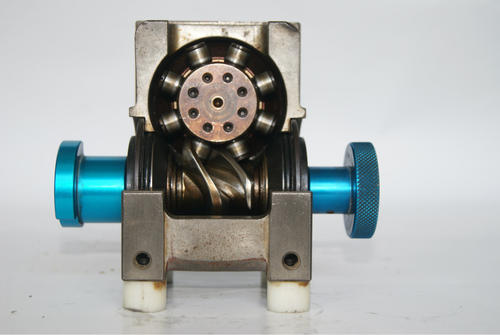分割器

# 凸轮分割器选型设计举例说明以下就是关于凸轮分割器的选型设计举例说明，已知条件:

(1)间歇分割定位等分:N=8 N:分割数

(2)每等分驱动时间:1/3秒 定位时间:2/3秒

(3)入力轴之回转数:n=60rpm:凸轮轴速度(第分钟转速)

(4)凸轮曲线:MS变形正弦曲线 (MT变形台型曲线 MS变形正弦曲线 MCV50变形等速度曲线)

(5)回转盘的尺寸:600×16T

(6)夹具的重量:2.5kg/组

(7)工件的重量:0.3kg/组

(8)转盘依*其底部的滑动面支持本身重量负荷,有效半径:R=250mm

(9)驱动角:θ=360×(驱动时间)/(驱动时间+定位时间)= 120 deg

选型详解: 问歇分割定位等分:N=8 输入轴的回转数:N=60rpm 凸输曲线是变形正弦曲线,因此Vm=1.76 Am=5.33 Om=0.99 负载扭矩:Tt

(1)惯性扭矩:Ti

(a) 转盘重量:w1夹盘重量;w2工件重量:w3则:

w1=πR2t×7.8×1/1000=π×302×1.6×7.8×1/1000=35.29(kg) w2=2.5×8×=20(kg) w3=0.3×8×=2.4(kg)

(b) 回转盘惯性矩:I1夹具惯性矩;I2工件惯性矩;I3为 I1=w1R2/2G =35.29×0.062/2×9.8=0.16(kg.m.s) I2= w2Re2/2G=20×0.252/2×9.8=0.13(kg.m.s) I3=w3R Re2/2G=20×0.252/2×9.8=0.015 (kg.m.s)

(c)总惯性矩:I i=I1+I2+I3 I=0.16+0.13+0.015=0.305(kg.m.s2)(1)静扭矩(惯性扭矩):Ti Ti=I×α=0.305×39.09=11.92(kg.m)

(2)磨擦扭矩:Tf Tf=μ×w×R=0.15×(35.29+20+2.4) ×0.25=2.16(Kg.m) --μ摩擦系数为0.15

(3)作功扭矩:Tw 在间歇分割时没有作功，因此Tw=0

(4)以上总负载扭矩:Tt Tt=Ti+Tf+Tw=11.92+2.16+0=14.08(kg.m)

实际负载扭矩:Te安全负载的因数fe=1.5 Te=Tt×fe=14.08×1.5=21.12(kg . m) --最终结果

输入轴扭矩:Tc注：输入轴起动负载扭矩视为Tca=0. 因此Tca=0 Tc=360/θh .s ×Qm×(Te+Tca)=360/(120×8) ×0.99×21.12=7.84(kg. m)

计算所需的马力:p=Tc×n / 716×Z(HP) 或 P=Tc×n / 975×Z (kw)

假设效率Z=60% 那么 P=7.84×60 / 716×0.6=1.09(HP)或P=7.84×60 / 975×0.6=0.84 (Kw) 事实上,以上所计算的值为起动时最大马力,而连续传动所需的马力为1/2 选择适用的间歇分割器根据以上所计算的资料以及输入轴的转数

60rpm来选择,请参考说明书上所记载,凡是输出轴扭矩高于以上所计算的Te值者均可选用。因为Te=21.12(kg-m),所以应采用DF110型。

Vm: 最大非向性速度 Am: 最大非向性之加速度 Qm: 凸轮轴最大扭力系数 α: 角加速度 θh : 输入轴转位(驱动) μ: 磨擦系数=0.15 γ: 速度比 Ω: 角速度(弧度/秒) α: 角加速度(弧度/秒2)